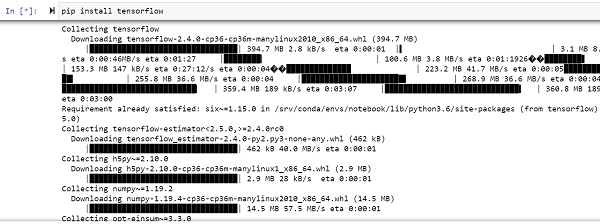# How can Tensorflow be used to multiply two matrices using Python?

Tensorflow is a machine learning framework that is provided by Google. It is an open−source framework used in conjunction with Python to implement algorithms, deep learning applications and much more. It is used in research and for production purposes. It has optimization techniques that help in performing complicated mathematical operations quickly.

This is because it uses NumPy and multi−dimensional arrays. These multi-dimensional arrays are also known as ‘tensors’. The framework supports working with deep neural network. It is highly scalable, and comes with many popular datasets. It uses GPU computation and automates the management of resources. It comes with multitude of machine learning libraries, and is well−supported and documented. The framework has the ability to run deep neural network models, train them, and create applications that predict relevant characteristics of the respective datasets.

The ‘tensorflow’ package can be installed on Windows using the below line of code −

pip install tensorflow

Tensor is a data structure used in TensorFlow. It helps connect edges in a flow diagram. This flow diagram is known as the ‘Data flow graph’. Tensors are nothing but multidimensional array or a list.

We will be using the Jupyter Notebook to run these code. TensorFlow can be installed on Jupyter Notebook using ‘pip install tensorflow’.Following is an example −

## Example

import tensorflow as tf
import numpy as np

matrix_1 = np.array([(1,2,3),(3,2,1),(1,1,1)],dtype = 'int32')
matrix_2 = np.array([(0,0,0),(-1,0,1),(3,3,4)],dtype = 'int32')
print("The first matrix is ")
print (matrix_1)
print("The second matrix is ")
print (matrix_2)
print("The product is ")
matrix_1 = tf.constant(matrix_1)
matrix_2 = tf.constant(matrix_2)
matrix_prod = tf.matmul(matrix_1, matrix_2)
print((matrix_prod))

## Output

The first matrix is
[[1 2 3]
[3 2 1]
[1 1 1]]
The second matrix is
[[ 0 0 0]
[−1 0 1]
[ 3 3 4]]
The product is
tf.Tensor(
[[ 7 9 14]
[ 1 3 6]
[ 2 3 5]], shape=(3, 3), dtype=int32)

## Explanation

• Import the required packages and provide an alias for it, for ease of use.

• Two matrices are created using the Numpy package.

• They are converted from being a Numpy array to a constant value in Tensorflow.

• The ‘matmul’ function in Tensorflow is used to multiply the values in the matrix.

• The resultant product is displayed on the console.